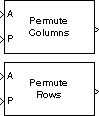# Permute Matrix

Reorder matrix rows or columns

•Libraries:

## Description

The Permute Matrix block reorders the rows or columns of an M-by-N input matrix `A` as specified by indexing input `P`.

## Ports

### Input

expand all

Input matrix, specified as a scalar, vector, or matrix.

Data Types: `single` | `double` | `int8` | `int16` | `int32` | `int64` | `uint8` | `uint16` | `uint32` | `uint64` | `Boolean` | `fixed point` | `enumerated`

Index matrix, specified as a scalar or vector.

Data Types: `single` | `double` | `int8` | `int16` | `int32` | `int64` | `uint8` | `uint16` | `uint32` | `uint64` | `Boolean` | `enumerated`

### Output

expand all

Output signal, specified as a vector or matrix.

Data Types: `single` | `double` | `int8` | `int16` | `int32` | `int64` | `uint8` | `uint16` | `uint32` | `uint64` | `Boolean` | `fixed point` | `enumerated`

## Parameters

expand all

Method of constructing the output matrix by permuting rows or columns of the input.

When the Permute parameter is set to:

• `Rows` –– The block uses the rows of `A` to create a matrix that has the same column dimension. Input `P` is a length-L vector whose elements determine where each row from `A` must be placed in the L-by-N output matrix.

`y = [A(P(1),:) ; A(P(2),:) ; A(P(3),:) ; ... ; A(P(end),:)] % Equivalent MATLAB code `

For row permutation, the block treats the length-M unoriented vector input at the port `A` as an M-by-1 matrix.

• `Columns` –– The block uses the columns of `A` to create a matrix that has the same row dimension. Input `P` is a length-L vector whose elements determine where each column from `A` must be placed in the M-by-L output matrix.

`% Equivalent MATLAB code y = [A(:,P(1)) A(:,P(2)) A(:,P(3)) ... A(:,P(end))] `

For column permutation, the block treats the length-N unoriented vector input at port `A` as a 1-by-N matrix.

#### Programmatic Use

 Block Parameter: `mode` Type: character vector, string Values:```' Columns'``` | `'Rows'` Default: ```' Columns'```

When set to `One-based`, a value of `1` in the permutation vector `P` refers to the first row or column of the input matrix `A`. When set to `Zero-based`, a value of `0` in `P` refers to the first row or column of `A`.

#### Programmatic Use

 Block Parameter: `ZeroOneIdxMode` Type: character vector, string Values:```' Zero-based'``` | `'One-based'` Default: `'One-based'`

Response to an invalid index value. When an index value in input `P` references a nonexistent row or column of matrix `A`, the block reacts as specified in this parameter. These options are available:

• `Clip index` –– Clip the index to the nearest valid value (1 or M for row permutation and 1 or N for column permutation) and do not issue an alert. Example: For a 3-by-7 input matrix, a column index of 9 is clipped to 7 and a row index of -2 is clipped to 1.

• `Clip and warn` –– Display a warning message in the MATLAB® Command Window and clip the index as described in the preceding bullet.

• `Generate error` –– Display an error dialog box and terminate the simulation.

Tunable: Yes

#### Programmatic Use

 Block Parameter: `errmode` Type: character vector, string Values: ```'Clip index'``` | `'Clip and warn'` | `'Generate error'` Default: ```'Clip index'```

Option to display an error dialog box and terminate the simulation when the length of the permutation vector `P` is not equal to the number of rows or columns of the input matrix `A`.

You can choose to open an error dialog box and terminate the simulation by setting this parameter to `on`.

#### Programmatic Use

 Block Parameter: `checkDims` Type: character vector, string Values: `'off'` | `'on'` Default: `'off'`

## Block Characteristics

 Data Types `Boolean` | `double` | `enumerated` | `fixed point` | `integer` | `single` Direct Feedthrough `no` Multidimensional Signals `no` Variable-Size Signals `no` Zero-Crossing Detection `no`

## Version History

Introduced before R2006a

expand all